### Home > MC1 > Chapter 2 > Lesson 2.2.3 > Problem2-80

2-80.

Each of the four diamonds below contains the same number pattern. Can you figure out what that pattern is?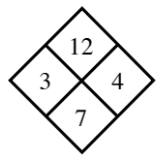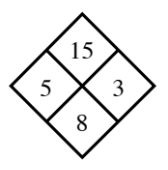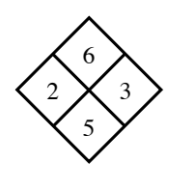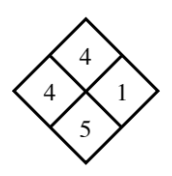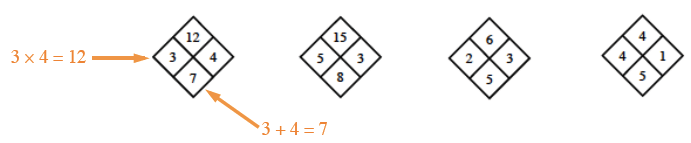Now use the pattern you found to copy and complete each of the Diamond Problems below.

1.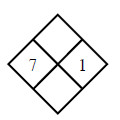This problem is solved like the ones above.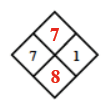1.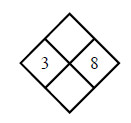This problem is solved like the ones above.

1.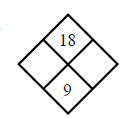Which two integers added together equal $9$ and multiplied together equal $18$?

1.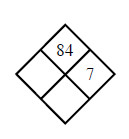What is $84$ divided by $7$?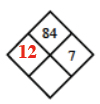Remember to complete the last box.